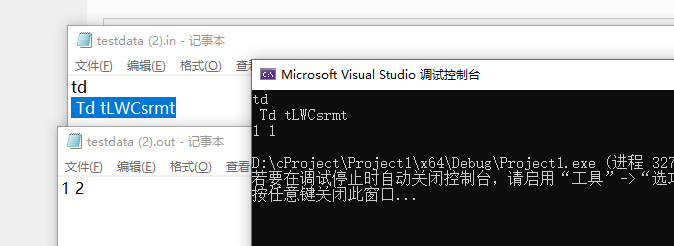# 本地运行是正确结果， 却和oj上下载来的错误输出文件内的数据不符。

@peroperotina 2019-11-10 04:23 回复

#include<stdio.h>
#include<string.h>
#include<ctype.h>
int main(void)
{
char vocabulary, string, * t;
int i = 0, position;
gets(vocabulary);
gets(string);
for (t = vocabulary; *t != '\0'; t++)
if (isalpha(*t))
*t = tolower(*t);
for (t = string; *t != '\0'; t++)
if (isalpha(*t))
*t = tolower(*t);
t = strstr(string, vocabulary);
if (t && (t == string || *(t - 1) == ' ') && (*(t + strlen(vocabulary)) == ' ' || *(t + strlen(vocabulary)) == '\0'))
{
position = strstr(string, vocabulary) - string;
i++;
while ((t=strstr(t + 1, vocabulary)))
i++;
printf("%d %d\n", position, i);
}
else
printf("-1\n");

return 0;
}@一个python屑 大佬吼啊